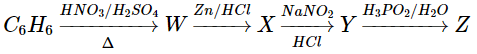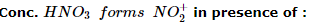Courses

# Test: Chemistry Mock- 4

## 45 Questions MCQ Test NEET Mock Test Series | Test: Chemistry Mock- 4

Description
This mock test of Test: Chemistry Mock- 4 for NEET helps you for every NEET entrance exam. This contains 45 Multiple Choice Questions for NEET Test: Chemistry Mock- 4 (mcq) to study with solutions a complete question bank. The solved questions answers in this Test: Chemistry Mock- 4 quiz give you a good mix of easy questions and tough questions. NEET students definitely take this Test: Chemistry Mock- 4 exercise for a better result in the exam. You can find other Test: Chemistry Mock- 4 extra questions, long questions & short questions for NEET on EduRev as well by searching above.
QUESTION: 1

Solution:
QUESTION: 2

Solution:
QUESTION: 3

### Catalysts used in cracking process are

Solution:
QUESTION: 4
Which of the following elements has least number of electrons in its M-shell
Solution:
QUESTION: 5

A solution of 10 mL of M/10 FeSO₄ was titrated with KMnO₄ solution in acidic medium, the amount of KMnO₄ used will be

Solution:
QUESTION: 6
Insulin, a hormone, chemically is
Solution:
QUESTION: 7
The shape of ClO₃⁻ according to valence shell electron repulsion theory will be
Solution:
QUESTION: 8

The heat of formation of the compound in the following reaction is
H₂(g) + Cl(g)→ 2HCl(g)+44 Kcal

Solution:
QUESTION: 9
The chemical equilibrium of a reversible reaction is not influenced by
Solution:
QUESTION: 10
For the reaction A + 2B → C, the rate of reaction at a given instant can be represented by
Solution:
QUESTION: 11
Which of the following is true for an isochoric processes
Solution:
QUESTION: 12
The dyes attached to the fibres themselves by irreversible chemical reactions are
Solution:
QUESTION: 13
The prefixes sys and anti are used to denote
Solution:
QUESTION: 14
The oxide of which of the following elements will be acidic in character
Solution: The oxides of metals are basic whereas the oxides of non-metals are acidic.
QUESTION: 15

Which of the following compounds is square planar and does not have any unpaired electron?

Solution:
QUESTION: 16
Which of the following is formed when KMnO₄ reacts with sodium thiosulphate?
Solution:
QUESTION: 17
Which is the best description of the behaviour of bromine in the reaction: H₂O + Br₂ → HOBr + HBr?
Solution:
QUESTION: 18
Which one among the following salts does not impart colour to the flame?
Solution:
QUESTION: 19
Which of the following statements is wrong for gases ?
Solution:
QUESTION: 20
Cs⁺ ion imparts violet colour to the flame. This is due to the fact that the emitted radiations have
Solution:
QUESTION: 21
In the electrolytic extraction of Aluminium fused cryolite is added. Its role is
Solution:
QUESTION: 22
Silicon is an important constituent of
Solution:
QUESTION: 23
Sodium hexametaphosphate is know as
Solution:
QUESTION: 24
When potassium ferrocyanide crystals are heated with conc.H₂SO₄, the gas evolved is
Solution:
QUESTION: 25
Bleaching powder is obtained by treating Cl₂ with
Solution:
QUESTION: 26
Plaster of Paris hardens by
Solution:
QUESTION: 27
Which of the following compounds liberates hydrogen gas on reacting with sodium metal?
Solution:
QUESTION: 28
The pH of H₂O₂ solution is 6.0. When some Cl₂ gas is bubbled through it,
Solution:
QUESTION: 29
Two solutions A and B are prepared by adding NaOH and HCI acid into water respectively. The ionic product of water will
Solution: Ionic product of water is always a constant at a particular temperature. It does not depend on any other things hence adding in solute cannot change the ionic product of water.
QUESTION: 30
The reason for geometrical isomerism by 2-butene is
Solution:
QUESTION: 31
Which responds carbylamine reaction?
Solution:
QUESTION: 32

'Z' in the following sequence of reactions isSolution:
QUESTION: 33
In reaction of alcohols with alkali metal, carboxylic acid etc., which of the following alcohol will react fastest?
Solution:
QUESTION: 34Solution:
QUESTION: 35
Ligands in a complex salt are
Solution:
QUESTION: 36
The most electropositive amongst the alkaline earth metals is
Solution: The electronegativity values for the elements decrease down a group. This is because as we move down the group atomic radius as well as screening effect increases. Thus, barium (Ba) is the least electronegative or most electropositive among the given alkaline earth metals.
QUESTION: 37
Glyptal polymer is obtained from glycerol on reacting with
Solution:
QUESTION: 38
If on adding FeCl₃ solution to acidified Lassaigne solution, a blood red colouration is produced, it indicates the presence of
Solution:
QUESTION: 39

Which is the strongest reducing agent ?

Solution:
QUESTION: 40
If the radius of an atom of an element is 75 pm and the lattice type is body-centred cube, then the edge length of the unit cell is
Solution:
QUESTION: 41

1.8 g of fructose (C₆H₁₂O₆) is added to 2kg of water. The freezing point of the solution is

Solution:
QUESTION: 42
Of the following compounds, which will have zero dipole moment?
Solution:
QUESTION: 43
The present atomic scale is based on
Solution:
QUESTION: 44
The process of separating a crystalloid from a colloid by filtration, is called
Solution:
QUESTION: 45
In the ground state, an element has 13 electrons in its "M shell". The element is.......
Solution: